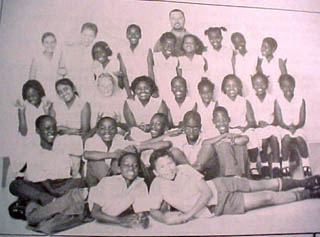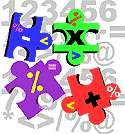Math Puzzlesfrom Botswana, Iceland and MarylandKgaswe Primary School - Palapye, Botswana From Daisy: I have a maths puzzle for you: this number is between 40 and 50. if you divide it by 5 you get a remainder of 3 if you divide it by 7 you get a remainder of 1 Hi Daisy, My name is Shelly and I found the answer to your problem. Is it 43? This is how I got the answer to your problem: I divide by 7 and 43/7 = 6 and a remainder of 1. 43/5 = 8 and a remainder of 3. Your friend, Shelly From Flora: I have got a maths puzzle for you , you will have to find the number here it goes. My number is between 50 and 55 it is a prime number and then you add 6, then subtract 15 what is my number? From Landi: Find my number. It is a number between 15 and 45. If you divide it by 4 you get a remainder of 1. If you times it by 8 the product will be 200. What is the numberFrom Michelle: This is a number puzzle try work it out ' how many wheels on a motor car + the letters of your name subtract 3 = write back and tell me the answer well I got to bounce good bye . Dear Michelle, Hello, my name is Melissa. I go to Delmar Elementary School. I saw a math puzzle that you made and I would like to try and figure it out. Well, here I go. Well you asked how many wheels on a car so 4. Then you asked how many letters are in my name and there are 7. So far it is 7+4=11. Now you -3. 11-3=8. Eight is the answer to the puzzle. Now see if you can figure out my math puzzle. How many letters in the English alphabet + how many numbers on a clock - the number of continents + the number of stars on the flag of the Federated States of Micronesia. What is your guess? A pal, Melissa C. From Shebo: I would like to make up a math puzzle for you to solve 1) A number between 9 and 24 if you divide it by 3 you get a remainder of 1 and if you divide it by 7 you get a remainder 2. What is the number? From Yvonne: There is one math puzzle I want to tell you and it is the number is between 20 and 36, if you divide it by it by four you get a remainder of one, if you divide it by 6 you get a remainder of 3. Hi! Yvonne ! I was able to solve your problem. It was a very fun problem to do. What I did was pick a number 20-36 and then divided it by 6 and got a remainder of 3 and then I check it by dividingr it by 4 and I got 1 as a remainder. So you answer to your problem is 33. Here is a problem for you! find out how many n's are in your name and then times it by a number between 20 and 30 then divide it by 5 when you divided it by 5 you will get 2 as a remainder. Well I can't wait to hear from you ! BYE!!!!!!!!!! Your Pal, Maranda From Danny: Here's a maths puzzle for you . Take the numbers of letters there in my first name , add the number of stars there is in the Stars and the Stripes.. From Shawn at Delmar: Hi Danny, Your Answer to your puzzle was 68. That was easy. Now here is one for you Take the number of letters of my first name time the number of fingers on your hand minus the number of brothers and sisters you have plus the number of animals you have. There is my math puzzle. Your Friend, Shawn Frye Hi Danny, Ops! I meant the answer was 55. I did not under stand what you said at first. I thought you meant the stars and strips put together. This is how I got my answer: Your name has 5 letters: D a n n y The Stars and Stripes has 50 stars and thirteen stripes but you didn't ask for stripes. I made a mistake and added the stripes. 50 + 5 = 55 Your Friend, Shawn Frye From Fjóla in Iceland Hć Patti, Am going to try to make a puzzle: My number is between 20 and 30. If I add 6 with her and draw 4 from her the number will be 20 what´s my number? All best Fjóla Hi Fjóla, Hello, my name is Melissa and I want to figure out your puzzle. Okay here I go. I don't think your number is between 20-30. I think your number is 18. The way I figured that was if you add 6 to 18 you get 24. Then you -4 from 24 and you get 20. Your pal, Melissa Cook from Fjóla in Iceland: Hello Patti Melissa was right the number was 18. can I make a pussle like this. Hello kids can you tell my how many colours are in the Icelandic flag if I add 6 with the number and then draw 3 from and it and then add 2 to the number again all get 8. Fjóla From Hulda in Iceland: My number is between 1-5. I will multiply the number with 3 and then I will get = 15 and then I will + with the number and then I will get =20 What is the number ????? Hulda | home | greetings | new | kids | teachers | visitors | search |Patricia A. Weeg author: Kids@work: Math in the Cyberzone page updated February 27, 2000